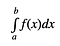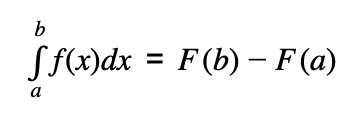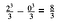# Computing Area Using Calculus

## A brief guide to finding area beyond squares, triangles, and circlesPhoto by Erlend Ekseth on Unsplash

How would you calculate the area of the circular region above? It’s simple enough. Anybody past the high school level should know that the area of a circle is just the radius squared multiplied by the magical constant pi. Similarly, we all know that the area of a square is just length times width, and the area of a triangle is just half of the previous expression. These are all equations we can rattle off without much thought. However, what happens when we are dealing with an area of a curved region? Then things get tricky.

# Introduction to Integrals

If you’ve taken calculus, this should be a nice trip down memory lane. But if you haven’t, what you’re about to see is truly amazing. To understand how to deal with areas involving a curve, we need to use something called an integral.

The integral is actually defined to be the net signed area under a curve (its value can be either positive or negative). This definition is derived from using a Reimann Sum, which is a limit. Essentially, what is happening is that we are dividing up the interval for which we are calculating the area into smaller bits that will serve as the base for rectangles under the curve.Screenshot on Desmos by Author

As you can see, if the length of the base of the rectangles is large, the estimation of the area is poor. The area becomes exact when the number of rectangles approaches infinity. This limit is defined as the integral of the function on a certain interval [a , b].Notation of an Integral

Now, how would we obtain the exact value of the area? To do this, we need to take advantage of the Fundamental Theorem of Calculus.

# The Theorem at WorkThe Fundamental Theorem of Calculus

The theorem states that the integral of f(x) on the interval [a , b] is equal to the antiderivative of f(x) evaluated at b minus the antiderivative of f(x) evaluated at a. To freshen up, the derivative of a function f(x) is another function f’(x) that measures the rate of change of the original function. The antiderivative of f(x) is just any function that when differentiated, will yield f(x). We generally note this as F(x) + C with the addition of a constant C, since the derivative of a constant is always 0. Now, we are ready to compute the exact value of the area under a curve.

Let’s use a simple example: find the area under the graph of f(x) = x² on the interval [0 , 2]. First we need to find the antiderivative of . Using the reverse power rule, we can easily show that this is x³/3 (yes, there is supposed to be a +C, but we can ignore that for these problems). Now, we need to evaluate this antiderivative at x = 2 and x = 0, and then compute the difference.Screenshot of Work by Author

# Conclusion

In this article, I briefly shared with you the logic and mechanics behind finding the area of irregular shapes. The main principle being used here is the Fundamental Theorem of Calculus, which helps us evaluate the area of strange functions on a certain interval. With this simple yet powerful idea, computing areas become a walk in the park. Hopefully, you’ll be able to impress your friends with this new trick!

If you have any questions, please feel free to leave them in the comment section.

## Math Simplified

Simplified is a publication aiming at making mathematics accessible and enjoyable.

Written by

## Albert Ming

17 || High School Junior || stats & math## Math Simplified

Simplified is a publication aiming at making mathematics accessible and enjoyable.

## More From Medium

Medium is an open platform where 170 million readers come to find insightful and dynamic thinking. Here, expert and undiscovered voices alike dive into the heart of any topic and bring new ideas to the surface. Learn more

Follow the writers, publications, and topics that matter to you, and you’ll see them on your homepage and in your inbox. Explore

If you have a story to tell, knowledge to share, or a perspective to offer — welcome home. It’s easy and free to post your thinking on any topic. Write on Medium